Articles tagged octave at null program 2023-03-24T14:55:58Z urn:uuid:ae7db2fb-eac3-4621-bae3-876c87011475 Christopher Wellons https://nullprogram.com wellons@nullprogram.com Perlin Noise With Octave, Java, and OpenCL urn:uuid:830cc950-634a-3661-135a-b932c8c5399e 2012-06-03T00:00:00Z I recently discovered that I’m an idiot and that my old Perlin noise post was not actually describing Perlin noise at all, but fractional Brownian motion. Perlin noise is slightly more complicated but much more powerful. To learn the correct algorithm, I wrote three different implementations (perlin-noise).

``````git clone git://github.com/skeeto/perlin-noise.git
``````

In short, Perlin noise is based on a grid of randomly-generated gradient vectors which describe how the arbitrarily-dimensional “surface” is sloped at that point. The noise at the grid points is always 0, though you’d never know it. When sampling the noise at some point between grid points, a weighted interpolation of the surrounding gradient vectors is calculated. Vectors are reduced to a single noise value by dot product.

Rather than waste time trying to explain it myself, I’ll link to an existing, great tutorial: The Perlin noise math FAQ. There’s also the original presentation by Ken Perlin, Making Noise, which is more concise but harder to grok.

When making my own implementation, I started by with Octave. It’s my “go to language” for creating a prototype when I’m doing something with vectors or matrices since it has the most concise syntax for these things. I wrote a two-dimensional generator and it turned out to be a lot simpler than I thought it would be!

Because it’s 2D, there are four surrounding grid points to consider and these are all hard-coded. This leads to an interesting property: there are no loops. The code is entirely vectorized, which makes it quite fast. It actually keeps up with my generalized Java solution (next) when given a grid of points, such as from `meshgrid()`.

The grid gradient vectors are generated on the fly by a hash function. The integer x and y positions of the point are hashed using a bastardized version of Robert Jenkins’ 96 bit mix function (the one I used in my infinite parallax starfield) to produce a vector. This turned out to be the trickiest part to write, because any weaknesses in the hash function become very apparent in the resulting noise.

Using Octave, this took two seconds to generate on my laptop. You can’t really tell by looking at it, but, as with all Perlin noise, there is actually a grid pattern.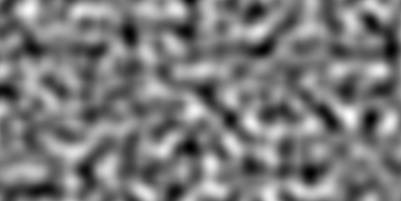I then wrote a generalized version, `perlin.m`, that can generate arbitrarily-dimensional noise. This one is a lot shorter, but it’s not vectorized, can only sample one point at a time, and is incredibly slow. For a hash function, I use Octave’s `hashmd5()`, so this one won’t work in Matlab (which provides no hash function whatsoever). However, it is a lot shorter!

``````%% Returns the Perlin noise value for an arbitrary point.
function v = perlin(p)
v = 0;
%% Iterate over each corner
for dirs = [dec2bin(0:(2 ^ length(p) - 1)) - 48]'
q = floor(p) + dirs'; % This iteration's corner
m = dot(g, p - q);
t = 1.0 - abs(p - q);
v += m * prod(3 * t .^ 2 - 2 * t .^ 3);
end
end

%% Return the gradient at the given grid point.
v = zeros(size(q));
for i = 1:length(q);
v(i) = hashmd5([i q]) * 2.0 - 1.0;
end
end
``````

It took Octave an entire day to generate this “fire” video, which is ridiculously long. An old graphics card could probably do this in real time.

This was produced by viewing a slice of 3D noise. For animation, the viewing area moves in two dimensions (z and y). One dimension makes the fire flicker, the other makes it look like it’s rising. A simple gradient was applied to the resulting noise to fade away towards the top.

I wanted to achieve this same effect faster, so next I made a generalized Java implementation, which is the bulk of the repository. I wrote my own Vector class (completely unlike Java’s depreciated Vector but more like Apache Commons Math’s RealVector), so it looks very similar to the Octave version. It’s much, much faster than the generalized Octave version. It doesn’t use a hash function for gradients — instead randomly generating them as needed and keeping track of them for later with a Map.

I wanted to go faster yet, so next I looked at OpenCL for the first time. OpenCL is an API that allows you to run C-like programs on your graphics processing unit (GPU), among other things. I was sticking to Java so I used lwjgl’s OpenCL bindings. In order to use this code you’ll need an OpenCL implementation available on your system, which, unfortunately, is usually proprietary. My OpenCL noise generator only generates 3D noise.

Why use the GPU? GPUs have a highly-parallel structure that makes them faster than CPUs at processing large blocks of data in parallel. This is really important when it comes to computer graphics, but it can be useful for other purposes as well, like generating Perlin noise.

I had to change my API a little to make this effective. Before, to generate noise samples, I passed points in individually to PerlinNoise. To properly parallelize this for OpenCL, an entire slice is specified by setting its width, height, step size, and z-level. This information, along with pre-computed grid gradients, is sent to the GPU.

This is all in the `opencl` branch in the repository. When run, it will produce a series of slices of 3D noise in a manner similar to the fire example above. For comparison, it will use the CPU by default, generating a series of `simple-*.png`. Give the program one argument, “opencl”, and it will use OpenCL instead, generating a series of `opencl-*.png`. You should notice a massive increase in speed when using OpenCL. In fact, it’s even faster than this. The vast majority of the time is spent creating these output PNG images. When I disabled image output for both, OpenCL was 200 times faster than the (single-core) CPU implementation, still spending a significant amount of time just loading data off the GPU.

And finally, I turned the OpenCL output into a video,

That’s pretty cool!

I still don’t really have a use for Perlin noise, especially not under constraints that require I use OpenCL to generate it. The big thing I got out of this project was my first experience with OpenCL, something that really is useful at work.

]]>
Lisp Let in GNU Octave urn:uuid:05e5318e-0cf4-3d80-4bf5-da695dbe9e47 2012-02-08T00:00:00Z In BrianScheme, the standard Lisp binding form `let` isn’t a special form. That is, it’s not a hard-coded language feature, or special form. It’s built on top of `lambda`. In any lexically-scoped Lisp, the expression,

``````(let ((x 10)
(y 20))
(* 10 20))
``````

Can also be written as,

``````((lambda (x y)
(* x y))
10 20)
``````

BrianScheme’s `let` is just a macro that transforms into a lambda expression. This is also what made it so important to implement lambda lifting, to optimize these otherwise-expensive forms.

It’s possible to achieve a similar effect in GNU Octave (but not Matlab, due to its flawed parser design). The language permits simple lambda expressions, much like Python.

``````> f = @(x) x + 10;
> f(4)
ans = 14
``````

It can be used to create a scope in a language that’s mostly devoid of scope. For example, I can avoid assigning a value to a temporary variable just because I need to use it in two places. This one-liner generates a random 3D unit vector.

``````(@(v) v / norm(v))(randn(1, 3))
``````

The anonymous function is called inside the same expression where it’s created. In practice, doing this is stupid. It’s confusing and there’s really nothing to gain by being clever, doing it in one line instead of two. Most importantly, there’s no macro system that can turn this into a new language feature. However, I enjoyed using this technique to create a one-liner that generates `n` random unit vectors.

``````n = 1000;
p = (@(v) v ./ repmat(sqrt(sum(abs(v) .^ 2, 2)), 1, 3))(randn(n, 3));
``````

Why was I doing this? I was using the Monte Carlo method to double-check my solution to this math problem:

What is the average straight line distance between two points on a sphere of radius 1?

I was also demonstrating to Gavin that simply choosing two angles is insufficient, because the points the angles select are not evenly distributed over the surface of the sphere. I generated this video, where the poles are clearly visible due to the uneven selection by two angles.

This took hours to render with gnuplot! Here are stylized versions: Dark and Light.

]]>
Silky Smooth Perlin Noise Surface urn:uuid:3b93a02f-93e1-3221-2405-58a83127968e 2012-01-19T00:00:00Z At work I’ve recently been generating viewsheds over DTED sets. Earlier this week I was asked to give an informal presentation on what I was doing. I wanted some terrain that demonstrated some key features, such as vision being occluded by hills of varying heights. Rather than search through the available DTED files for something good, I opted for generating my own terrain, using an old trick of mine: my noise “cloud” generator. That’s a lesson in the usefulness of maintaining a blog. The useful things you learn and create are easy to revisit years later!

I generated some noise, looked at it with `surf()`, and repeated until I found something useful. (Update June 2012: the function is called `perlin()` but it’s not actually Perlin noise.)

``````m = perlin(1024);
surf(m);
``````

The generated terrain is really quite rough, so I decided to smooth it out by convolving it with a 2-dimensional Gaussian kernel.

``````k = fspecial('gaussian', 9);
ms = conv2(m, k, 'same');
``````

It still wasn’t smooth enough. So I repeated the process a bit,

``````for i = 1:10
ms = conv2(ms, k, 'same');
end
``````

Perfect! I used that for my presentation. However, I was having fun and decided to experiment more with this. I filtered it again another 1000 times and generated a `surf()` plot with a high-resolution colormap — the default colormap size caused banding.

``````colormap(copper(1024));
surf(ms, 'EdgeAlpha', 0);
axis('equal');
``````

It produced this beautiful result!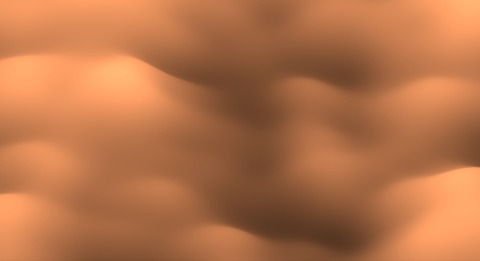I think it looks like a photograph from a high-powered microscope, or maybe the turbulent surface of some kind of creamy beverage being stirred.

At work when I need something Matlab-ish, I use Octave about half the time and Matlab the other half. In this case, I was using Matlab. Octave doesn’t support the `EdgeAlpha` property, nor the `viewshed()` function that I needed for my work. Matlab currently makes much prettier plots than Octave.

]]>
A GNU Octave Feature urn:uuid:a8aec192-263a-38d3-5d0d-86824c93fd4f 2008-08-29T00:00:00Z

At work they recently moved me to a new project. It is a Matlab-based data analysis thing. I haven't really touched Matlab in over a year (the last time I used Matlab at work), and, instead, use GNU Octave at home when the language is appropriate. I got so used to Octave that I found a pretty critical feature missing from Matlab's implementation: treat an expression as if it were of the type of its output.

Let's say we want to index into the result of a function. Take, for example, the magic square function, `magic()`. This spits out a magic square of the given size. In Octave we can generate a 4x4 magic square and chop out the middle 2x2 portion in one line.

```octave> magic(4)(2:3,2:3)
ans =

11   10
7    6
```

Or more possibly clearly,

```octave> [magic(4)](2:3,2:3)
ans =

11   10
7    6
```

Try this in Matlab and you will get a big, fat error. You have to assign the magic square to a temporary variable to do the same thing. I kept trying to do this sort of thing in Matlab and was thinking to myself, "I know I can do this somehow!". Nope, I was just used to having Octave.

Where this really shows is when you want to reshape a matrix into a nice, simple vector. If you have a matrix `M` and want to count the number of NaN's it has, you can't just apply the `sum()` function over `isnan()` because it only does sums of columns. You can get around this with a special index, `(:)`.

So, to sum all elements in `M` directly,

```octave> sum(M(:))
```

In Octave, to count NaN's with `isnan()`,

```octave> sum(isnan(M)(:))
```

Again, Matlab won't let you index the result of `isnan()` directly. Stupid. I guess the Matlab way to do this is to apply `sum()` twice.

Every language I can think of handles this properly. C, C++, Perl, Ruby, etc. It is strange that Matlab itself doesn't have it. Score one more for Octave.

]]>
Linear Spatial Filters with GNU Octave urn:uuid:e3b9e7a9-5669-3173-f84d-d8af0d8f8be4 2008-02-22T00:00:00Z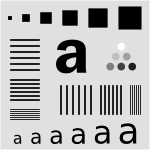I have gotten several e-mails lately about using GNU Octave. One specifically was about blurring images in Octave. In response, I am writing this in-depth post to cover spatial filters, and how to use them in GNU Octave (a free implementation of the Matlab programming language). This should be the sort of information you would find near the beginning of an introductory digital image processing textbook, but written out more simply. In the future, I will probably be writing a post covering non-linear spatial and/or frequency domain filters in Octave.

If you want to follow along in Octave, I strongly recommend that you upgrade to the new Octave 3.0. It is considered stable, but differs significantly from Octave 2.1, which many people may be used to. You will also need to install the image processing package from Octave-Forge. To get help with any Octave function, just type ```help <function>```.

The most common linear spatial image filtering involves convolving a filter mask, sometimes called a convolution kernel, over an image, which is a two-dimensional matrix. In the case of an RGB color image, the image is actually composed of three two-dimensional grayscale images, each representing a single color, where each is convolved with the filter mask separately.

Convolution is sliding a mask over an image. The new value at the mask's position is the sum of the value of each element of the mask multiplied by the value of the image at that position. For an example, let's start with 1-dimensional convolution. Define a mask,

```5 3 2 4 8
```

The 2 is the anchor for the mask. Define an image,

```0 0 1 2 1 0 0
```

As we convolve, the mask will extend beyond the image at the edges. One way to handle this is to pad the image with 0's. We start by placing the mask at the left edge. (zero-padding is underlined)

```Mask:   5 3 2 4 8
Image:  0 0 0 0 1 2 1 0 0
```

The first output value is 8, as every other element of the mask is multiplied by zero.

```Output: 8 x x x x x x
```

Now, slide the mask over by one position,

```Mask:   5 3 2 4 8
Image:  0 0 0 1 2 1 0 0
```

The output here is 20, because 8*2 + 4*1 = 20;

```Output: 8 20 x x x x x
```

If we continue sliding the mask along, the output becomes,

```Output: 8 20 18 11 13 13 5
```

Here is the correlation done in Octave interactively, (`filter2()` is the correlation function).

```octave> filter2([5 3 2 4 8], [0 0 1 2 1 0 0])
ans =

8   20   18   11   13   13    5

```

The same thing happens in two-dimensional convolution, with the mask moving in the vertical direction as well, so that each element in the image is covered.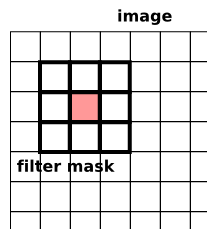Sometimes you will hear this described as correlation (Octave's `filter2`) or convolution (Octave's `conv2`). The only difference between these operations is that in convolution the filter masked is rotated 180 degrees. Whoop-dee-doo. Most of the time your filter is probably symmetrical anyway. So, don't worry much about the difference between these two. Especially in Octave, where rotating a matrix is easy (see `rot90()`).

Now that we know convolution, let's introduce the sample image we will be using. I carefully put this together in Inkscape, which should give us a nice scalable test image. When converting to a raster format, there is a bit of unwanted anti-aliasing going on (couldn't find a way to turn that off), but it is minimal.

Save that image (the PNG file, not the linked SVG file) where you can get to it in Octave. Now, let's load the image into Octave using `imread()`.

```m = imread("image-test.png");
```

The image is a grayscale image, so it has only one layer. The size of `m` should be 300x300. You can check this like so (note the lack of semicolon so we can see the output),

```size(m)
```

You can view the image stored in `m` with `imshow`. It doesn't care about the image dimensions or size, so until you resize the plot window, it will probably be stretched.

```imshow(m);
```

Now, let's make an extremely simple 5x5 filter mask.

```f = ones(5) * 1/25
```

Octave will show us what this matrix looks like.

```f =

0.040000   0.040000   0.040000   0.040000   0.040000
0.040000   0.040000   0.040000   0.040000   0.040000
0.040000   0.040000   0.040000   0.040000   0.040000
0.040000   0.040000   0.040000   0.040000   0.040000
0.040000   0.040000   0.040000   0.040000   0.040000
```

This filter mask is called an averaging filter. It simply averages all the pixels around the image (think about how this works out in the convolution). The effect will be to blur the image. It is important to note here that the sum of the elements is 1 (or 100% if you are thinking of averages). You can check it like so,

```sum(f(:))
```

Now, to convolve the image with the filter mask using `filter2()`.

```ave_m = filter2(f, m);
```

You can view the filtered image again with `imshow()` except that we need to first convert the image matrix to a matrix of 8-bit unsigned integers. It is kind of annoying that we need this, but this is the way it is as of this writing.

```ave_m = uint8(ave_m);
imshow(ave_m);
```

Or, we can save this image to a file using `imwrite()`. Just like with `imshow()`, you will first need to convert the image to `uint8`.

```imwrite("averaged.png", ave_m);
```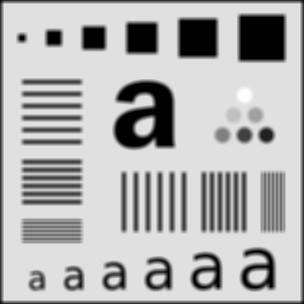There are a few things to notice about this image. First there is a black border around the outside of the filtered image. This is due to the zero-padding (black border) done by `filter2()`. The border of the image had 0's averaged into them. Second, some parts of the blurred image are "noisy". Here are some selected parts at 4x zoom.Notice how the circle, and the "a" seem a little bit boxy? This is due to the shape of our filter. Also notice that the blurring isn't as smooth as it could be. This is because the filter itself isn't very smooth. We'll fix both these problems with a new filter later.

First, here is how we can fix the border problem: we pad the image with itself. Octave provides us three easy ways to do this. The first is replicate padding: the padding outside the image is the same as the nearest border pixel in the image. Circular padding: the padding from from the opposite side of the image, as if it was wrapped. This would be a good choice for a periodic image. Last, and probably the most useful is symmetric: the padding is a mirror reflection of the image itself.

To apply symmetric padding, we use the `padarray()` function. We only want to pad the image by the amount that the mask will "hang off". Let's pad the original image for a 9x9 filter, which will hang off by 4 pixels each way,

```mpad = padarray(m, [4 4], "symmetric");
```

Next, we will replace the averaging filter with a 2D Gaussian distribution. The Gaussian, or normal, distribution has many wonderful and useful properties (as a statistics professor I had once said, anyone who considers themselves to be educated should know about the normal distribution). One property that makes it useful is that if we integrate the Gaussian distribution from minus infinity to infinity, the result is 1. The easiest way to get the curve without having to type in the equation is using `fspecial()`: a special function for creating image filters.

```f_gauss = fspecial("gaussian", 9, 2);
```

This creates a 9x9 Gaussian filter with variance 2. The variance controls the effective size of the filter. Increasing the size of the filter from 9 to 99 will actually have virtually no impact on the final result. It just needs to be large enough to cover the curve. Six times the variance covers over 99% of the curve, so for a variance of 2, a filter of size 7x7 (always make your filters odd in size) is plenty. A larger filter means a longer convolution time. Here is what the 9x9 filter looks like,And to filter with the Gaussian,

```gauss_m = filter2(f_gauss, mpad, "valid";
gauss_m = uint8(guass_m);
```

Notice the extra argument `"valid"`? Since we padded the image before filtering, we don't want this padding to be part of the image result. `filter2()` normally returns an image of the same size as the input image, but we only want the part that didn't undergo (additional) zero-padding. The result is now the same size as the original image, but without the messy border,Also, compare the result to the average filter above. See how much smoother this image is? If you are interested in blurring an image, you will generally want to go with a Gaussian filter like this.

Now I will let you in on a little shortcut. In Matlab, there is a function called `imfilter` which does the padding and filtering in one step. As of this writing, the Octave-Forge image package doesn't officially include this function, but it is there in the source repository now, meaning that it will probably appear in the next version of that package. I actually wrote my own before I found this one. You can grab the official one here: imfilter.m

With this new function, we can filter with the Gaussian and save like this. Notice the flipping of the first two arguments from `filter2`, as well as the lack of converting to `uint8`.

```gauss_m = imfilter(m, f, "symmetric");
imwrite("gauss.png", gauss_m);
```

`imfilter()` will also handle the 3-layer color images seamlessly. Without it, you would need to run `filter2()` on each layer separately.

So that is just about all there is. `fspecial()` has many more filters available including motion blur, unsharp, and edge detection. For example, the Sobel edge detector,

```octave:25> fspecial("sobel")
ans =

1   2   1
0   0   0
-1  -2  -1
```

It is good at detecting edges in one direction. We can rotate this each way to detect edges all over the image.

```mf = uint8(zeros(size(m)));
for i = 0:3
mf += imfilter(m, rot90(fspecial("sobel"), i));
end
imshow(mf)
```Happy Hacking with Octave!

]]>
Noise Fractals and Clouds urn:uuid:bbf7bad8-c18d-35b8-c7fc-da4fe43944e0 2007-11-20T00:00:00Z I was reading about fractal terrains and wanted to give it a shot. As usual, I like to try things out in Octave first by writing up a quick prototype, and, if it is still interesting enough, I will move to another language for better performance (Octave can be frustratingly slow sometimes). Additionally, when it comes to matrix manipulation, the Matlab language tends to be the most concise and powerful. Most functions and operators work on entire matrices or vectors at a time, avoiding many iteration loops, and, more notably, matrix notation built right into the language. This is powerful in the same way regular expressions are built into Perl. The problem is when your data shouldn’t be in matrix form, and the language, lacking any other kinds of data structures (especially hash tables!), forces you to fit your problem into matrices. Also, the implementations of the Matlab language tend to be very slow.

Anyway, back to noise.

So, the first and easiest noise algorithm I found was the diamond-square algorithm. Basically, it is noisy interpolation applied recursively. All of the noise adds up to provide something that may be good for height maps, possibly providing procedurally generated terrain for a game. Here is an example/pretty picture. Imagine this as being terrain,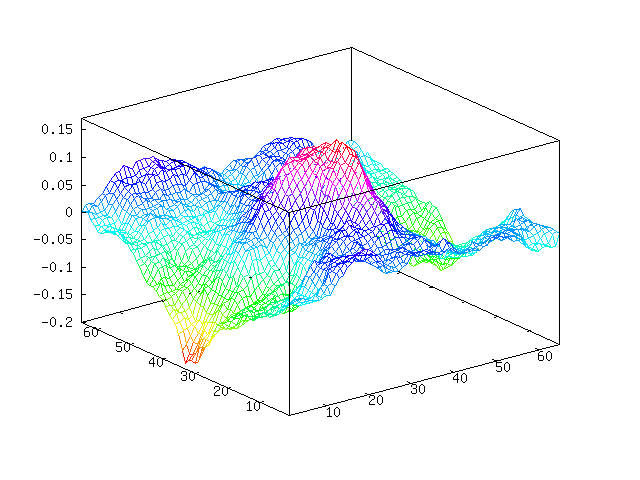You can see we have a sort of mountain going on in the middle. Here is the “plasma fractal” view of our noise.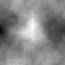These were generated using this Octave code. I believe that this is both the fastest and most concise way to do this in Octave. You can see we are throwing away a lot of random values that we pulled, but at the same time avoiding loops. You will need at least version 2.9 of Octave because, as far as I know, Octave 2.1 doesn’t have `interp2`. And, unfortunately, `interp2` is much slower than it probably could be.

``````function m = diamond_square (m, i, c)
if isempty (m)
m = zeros (2);
end

for k = 1:i
m = interp2 (m, 1);

%% Define points we want to randomize
gridmap = ones (size (m));
gridmap(1:2:end, 1:2:end) = 0;
gridmap = find(gridmap);       % Makes Octave happy

%% Define random values to be added
randmap = c * randn (size (m));

m(gridmap) += randmap(gridmap);

c = c / 2;
end
end
``````

Something of note here compared to other implementations of diamond-square, my code above adds Gaussian noise (with Octave’s `randn`) rather than uniform noise. The scaling variable `c` is actually adjusting the standard deviation of our noise and not the limits of the noise. I imagine this makes the terrain more natural, as Gaussian noise tends to approximate real noise well.

The first argument provides a base terrain to work from. With this you can define a mountain, islands, hillside, etc. When an empty matrix is provided, the terrain will be grown from flat ground. The second argument decides how many iterations we are going to run. The above example performs 6 iterations on flat terrain. The last argument decides how dramatic the terrain is, which doesn’t have much meaning when starting from flat terrain. The example above was produced with two calls like this (one for each image),

``````octave> mesh(m=diamond_square([], 6, 0.1))
octave> imagesc(m); colormap(gray);
``````

We can add some water, or perhaps lava or something, by choosing a water height and chopping off everything below it. I will choose `-0.1` as our water level.

``````octave> w = m;
octave> w(w &lt; -0.01) = -0.01;
octave> mesh(w)
``````Here is an example of providing some base terrain. Let’s put a mountain in the corner of the map,

``````octave> a = [0 0 0 0 0; 0 0 0 0 0; 0 0 0 0 0; 0 0 0 1 0; 0 0 0 0 0]
octave> mesh (b = diamond_square (a, 4, 0.15))
``````Becomes,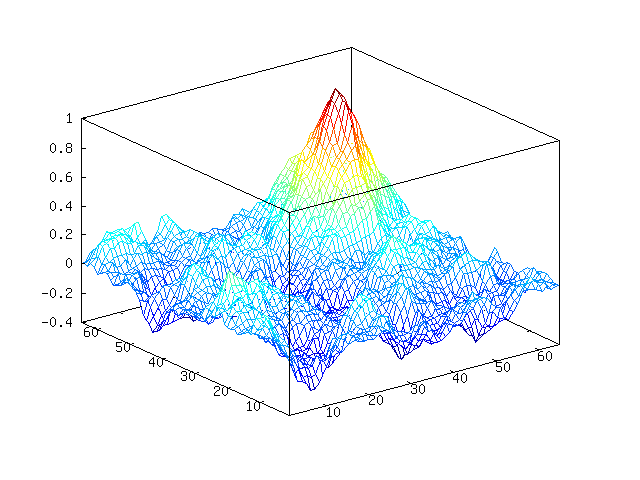Octave-Forge has a `surf` function that should make this look nicer, but it doesn’t. If we want to render this to make it look nice, we will need another program to do it. Let’s do that another time.

Update May 2012: I have come to realize the below is not Perlin noise. It is actually fractional Brownian motion, which is a bit simpler than Perlin noise.

Another way to make noise is fractional Brownian motion (FBM). Generally, this noise will be better and more useful than diamond-square noise (examples later). I personally like this person’s (mislabeled) approach: FPM Noise. It is easy to implement and understand, though it doesn’t have the all advantages you get from generating Perlin noise.

If you don’t feel like clicking through and see the nice introduction to FBM noise there, here is the idea of this approach: we create different frequencies of noise and mix them all together, giving more weight to lower frequency noise than high frequency noise. Below, you can see that I generated (Gaussian) noise in different frequencies, smoothing by spline interpolation. Then we add this all together to get our final FBM noise.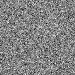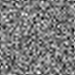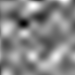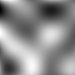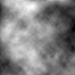Here is the Octave code I am using to generate FBM noise,

``````function s = fbm (m)
s = zeros(m);    % output image
w = m;           % width of current layer
i = 0;           % iterations
while w > 3
i = i + 1;
d = interp2(randn(w), i-1, "spline");
s = s + i * d(1:m, 1:m);
w -= ceil(w/2 - 1);
end
end
``````

The first and only argument gives the side length of the image you want to generate - it only generates square images. This is an extremely simple approach, as there are no parameters to adjust to define the nature of the noise you want to generate. Fortunately, what I am going to try next works fine without these parameters (or with the default, hard-coded parameters if you wish).

Continuing with using some ideas from Hugo Elias, I am going to use this noise to attempt to create some realistic looking clouds.

First, we will generate some noise. Let’s generate some FBM noise,

``````octave> n = fbm (200);
``````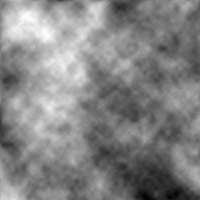Now, this doesn’t look too much like clouds. To get clouds, we can apply an exponential function to the data and set anything below 0 to 0 (contrast stretching). If we scale our noise between 0 and 1, the function will look like this,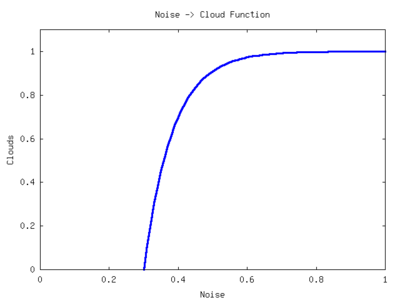To adjust the clouds, we can move this function left and right across the x-axis. We can adjust the “time constant” of the function to change the sharpness of the clouds. Here is the function I wrote to convert the diamond-square or FBM noise into cloud cover. The output is scaled between 0 and 255 to aid in image output.

``````function a = get_clouds (a)
%% Scale a between 0 and 1
a = a - min(a(:));
a = a / max(a(:));

%% Parameters
density = 0.5;
sharpness = 0.1;

a = 1 - e.^(-(a - density) * sharpness);
a(a < 0) = 0;

%% Scale between 0 to 255 and quantize
a = a / max(a(:));
a = round(a * 255);
end
``````

Now run this on our noise,

``````octave> c = get_clouds(n);
``````Well, that looks a bit more like cloud cover. We just need to apply a colormap to this. I wrote this colormap function for this purpose,

``````function c = cloud_cmap ()
c = [0.25 0.25 1];
for i = 2:256
c(i, :) = (i-2)/256 * (1 - c(2, :)) + c(2, :);
end
end
``````

Apply the colormap,

``````octave> imwrite("clouds.png", c+1, cloud_cmap)
``````Wow, that looks pretty good now. We could improve this by fading to transparent rather than blue. Then do a projective transformation on the clouds and lay them over top a blue gradient. You could do this with image editing software such as the Gimp, or you can continue to use Octave like I did at the end of this post.

So, in about 30 lines of Octave (including code on the interactive command line) code we could generate some kind-of realistic looking clouds. Here, I will use the cloud demo to show one particular advantage of FBM noise over diamond-square (or at least my implementation). Here are some diamond-square clouds,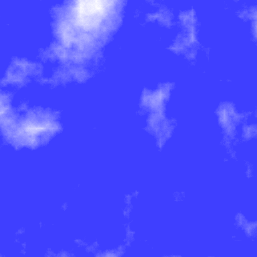And here are some FBM clouds, which I think look a bit better,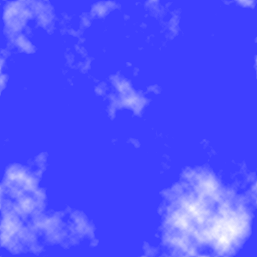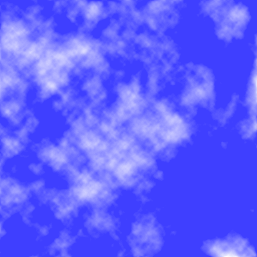Notice the straight lines in the diamond-square clouds? You can see it right in the middle of the first image. This comes from the way that the 2-dimensional interpolation stretches the noise in the vertical and horizontal directions, drawing these lines out. This may only be apparent in my implementation, as I am probably missing the “diamond” part of the algorithm. Oh well.

Anyway, to take the clouds a bit further, you can use Octave’s `imperspectivewarp` to apply a perspective transformation to the cloud images. I put some code together that does this transformation as well as adds a gradient,

``````function i = pers_clouds (n)
w = size(n, 1);
c = get_clouds (n);
t = -pi/32;
P = [cos(t) sin(t) 0; -sin(t) cos(t) 0; 0.001 0.002 1];

%% Perspective transformation
pc = imperspectivewarp (c/255, P, "cubic");
pw = size(pc, 1);
ph = size(pc, 2);

%% Create and combine background gradient
[dump back] = meshgrid(1:ph, 1:pw);
i = pc * 4 * pw + back;

%% Fit between 0 to 255 for image
i = i - min (i(:));
i = round (i / max (i(:)) * 255);
i(isnan(i)) = 0;
end
``````

Provide either FBM noise or diamond-square noise and it will return an image that you can write out to a file,

``````octave> n = fbm (1000);
octave> imwrite ("clouds.png", pers_clouds(n) + 1, cloud_cmap)
``````

After cropping the image with something like kolourpaint (as I did below), you get,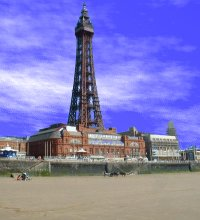Update: Sebastian Schaetz used my code above in a random map generator. Check it out.

]]>
Walk Text Adventure Game urn:uuid:3d597eab-e922-3eca-1a47-6211b34b9a9b 2007-09-25T00:00:00Z I wrote this thing back in September 2005 when I had to learn Matlab for work. This writeup was done about year later.

This was a text-adventure engine I wrote when I was starting to learn Matlab programming, which means the Matlab code here is pretty horrendous. It may or may not work in GNU octave, I didn’t bother checking. That’s because I later wrote a Perl version that does the same thing (when I was still starting with learning Perl, so not much better). Included is a sample “world” to explore (in English and pig latin!). The format for the “world” is a really simple one, probably missing a lot of features of a real text adventure scripting language. Have fun!

This is what it looks like,

``````Welcome to Walk. Just type in plain English into the 'Action:' line what you
want to do.

Type 'inv' to display the inventory.
Type 'clc' to clear the screen
Type 'look' or 'ls' to get the current description of the room.
Type 'save' to quicksave the session.
Type 'load' to restore the quicksave session.
Type 'doc' or 'help' to see this message again.
Type 'quit' to quit.

Action: look

You appear to be in a small bedroom. There are no windows. Light is
provided by a small ceiling lamp. There is a brown wooden door, a small bed
with no sheets, a small box which is laying on the bed.

Action: _
``````
]]>
Mandelbrot with GNU Octave urn:uuid:a8de095a-46bf-3835-5cff-68c91186ca80 2007-09-02T00:00:00Z In preparation for another project idea I have (to be posted in the future), I wrote a Mandelbrot fractal generator in Octave. Octave is great for just trying things out and prototyping your algorithms. It is very slow, however. Here is the code,

``````function mandel_img = mandel ()
%% Parameters
w = [-2.5 1.5]; % Domain
h = [-1.5 1.5]; % Range
s = 0.005;      % Step size
it = 64;        % Iteration depth

%% Prepare the complex plane
[wa ha] = meshgrid (w(1):s:w(2), h(1):s:h(2));
complex_plane = wa + ha * i;

%% Preallocate image
mandel_img = zeros( length(h(1):s:h(2)), length(w(1):s:w(2)));

%% Generate mandelbrot
for wi = 1:size(mandel_img, 2)
for hi = 1:size(mandel_img, 1)

z = 0;
k = 0;
while k < it && abs(z) < 2
z = z^2 + complex_plane (hi, wi);
k = k + 1;
end
mandel_img (hi, wi) = k - 1;

end
%% Display progress
waitbar (wi/size(mandel_img, 2));
end
end
``````

You may need to comment out the `waitbar` line if you do not have Octave-Forge installed properly (as is the case with Octave 2.9 on Debian as of this writing) or at all. You will also need Octave-Forge if you want to use the image functions described below. (This information is out of date.)

You can find the same code all over the Internet for many different languages. The advantage with Octave is that it knows about complex numbers so that this can be expressed directly with `z = z^2 + c` and `abs(z)`.

Now, this code just generates a matrix of the escape iteration numbers for each pixel. To visualize this, you will need to use the image functions. The simplest thing to do is view the data as a boring greyscale image.

``````octave> m = mandel(); # Generate the data
octave> imshow(m);
``````

You should see something like this,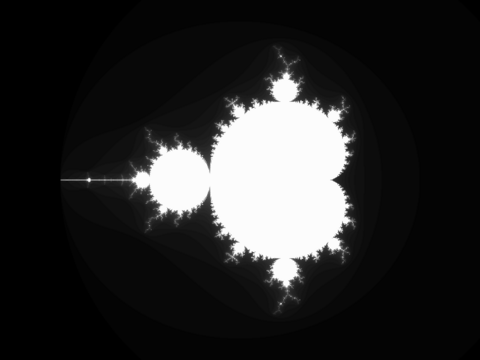You can save this as an image with `imwrite`,

``````octave> imwrite("mandel.png", m*4)
``````

The `*4` part is because the iteration depth was set to 64. The image being written will have values between 0 and 255. This allows the data to use the full dynamic range of the image.

If you want more interesting images, you can apply a colormap. Octave-Forge has two handy color maps, `hot` and `ocean` (cool). To make the inside of the fractal black, which are the points that are part of the set and never escaped, stick black on the end of the colormap. This can be done like this (viewing and saving),

``````octave> cmap = [hot(63); 0 0 0];  # The colormap
octave> imshow(m + 1, cmap);
octave> imwrite("mandel.png", m + 1, cmap);
```````m` is between 0 and 63. We add one to it to put it between 1 and 64. Then we take the colormap of length 63 and stick black on the end. If you substitute `ocean` for `hot`, you will get a nice blue version.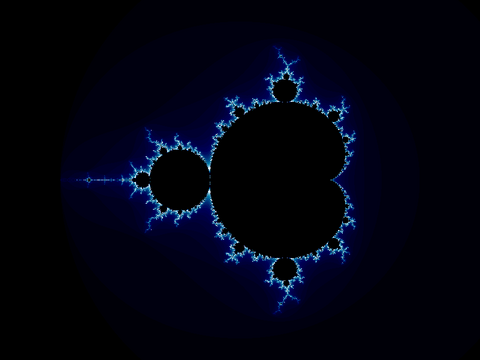You can modify the code above to try to get different fractals. For example, try `z = z^4 + c` instead,More on fractals another time.

]]>